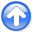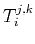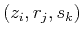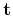First-break traveltime tomography with the double-square-root eikonal equationNext: Bibliography Up: Li et al.: DSR Previous: Appendix B: Frechét derivative

# Appendix C: Adjoint-state tomography with upwind finite-differences

Following Appendix A, we letin the DSR case be the traveltime at vertexand approximatein equation 8 by a one-sided finite-difference(41)

where thesign corresponds to the two neighbors ofindirection. An upwind scheme (Franklin and Harris, 2001) picks the sign by(42)

The above strategy can be applied toandstraightforwardly. For the shot-indexed eikonal equation 9, we approximatewith the same upwind method whilein this case is indexed forand.

For a Cartesian ordering of the discretized, i.e. vector, the discretized operatorswithare matrices. Thanks to upwind finite-differences, these matrices are sparse and contain only two non-zero entries per row. For instance, supposehas its upwind neighbor inat, then(43)

where(44)

Definitions of,andfollow their upwind approximations, respectively. In equation C-3,are located in the same row as that ofin. Whilesits on the diagonal,has a column index equals to the row ofin. At, there is no upwind neighbor and the corresponding row contains all zeros.

We can sort entries ofby their values in an increasing order, which equivalently performs column-wise permutations to. The results are lower triangular matrices. In fact, during FMM forward modeling, such an upwind ordering is maintained and updated by the priority queue and thus can be conveniently imported for usage here.

Note that the summation and subtraction of two (or more)matrices are still lower triangular. These matrices are also invertible, except for a singularity atwhere we may set the entries to be zero. Naturally, the inverted matrices are also lower triangular. One example is the linearized eikonal equation that gives rise to equation 10. Following notation C-4 and assuming the upwind neighbors ofareand, the linearized equation 9 reads(45)

After regrouping the terms, we get(46)

Equation C-6 means the inverse of the operatordoes not need to be computed by an explicit matrix inversion. Instead, we can perform its application to a vector by a single sweep based on causal upwind ordering. The same conclusion can be drawn for operator B-4.

Lastly, the adjoint-state calculations implied by equations 12 and 16 multiply the transpose of these inverse matrices with the data residual. The matrix transposition leads to upper triangular matrices. Accordingly, we solve the linear system with anti-causal downwind ordering that follows a decrease in values of.First-break traveltime tomography with the double-square-root eikonal equationNext: Bibliography Up: Li et al.: DSR Previous: Appendix B: Frechét derivative

2013-10-16